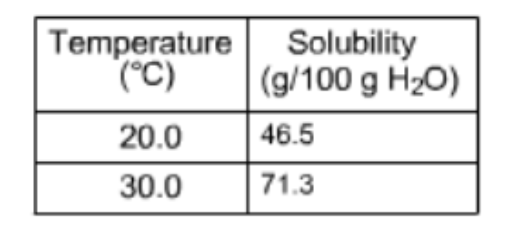# Problem: Here are the solubilities of a particular solute at two different temperatures. Suppose that you have made a saturated solution of this solute using 53.0 g of water at 20.0 °C. How much more solute can you add if the temperature is increased to 30.0 C?

91% (59 ratings)
###### FREE Expert Solution
91% (59 ratings)###### Problem Details

Here are the solubilities of a particular solute at two different temperatures. Suppose that you have made a saturated solution of this solute using 53.0 g of water at 20.0 °C. How much more solute can you add if the temperature is increased to 30.0 C?What scientific concept do you need to know in order to solve this problem?

Our tutors have indicated that to solve this problem you will need to apply the Henry's Law concept. You can view video lessons to learn Henry's Law. Or if you need more Henry's Law practice, you can also practice Henry's Law practice problems.

What is the difficulty of this problem?

Our tutors rated the difficulty ofHere are the solubilities of a particular solute at two diff...as medium difficulty.

How long does this problem take to solve?

Our expert Chemistry tutor, Sabrina took 3 minutes and 2 seconds to solve this problem. You can follow their steps in the video explanation above.

What professor is this problem relevant for?

Based on our data, we think this problem is relevant for Professor Faust's class at WOOSTER.